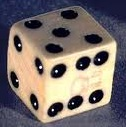# Complementary Events

Probability > Complementary events

## What are Complementary Events?

Complementary events happen when there are only two outcomes, like getting a job, or not getting a job. In other words, the complement of an event happening is the exact opposite: the probability of it not happening.

Examples of Complementary Events

• It rains or it does not rain.
• You pass your class or you don’t.
• Your dog bites the mailman, or it doesn’t.
• You win the lotto, or you don’t.
• You get married, or you don’t get married.The odds do not have to be equal. The important thing is that the two probabilities (the probability of an event happening and the probability of it not happening) must add up to 1. Rolling a die to see if you would get six is also complementary; the only two outcomes are: getting a six (a 1/6 chance) or not getting a six (5/6 chance). The odds of you winning the lotto might be one in a million; your odds of not winning then are 999,999 out of a million. The two probabilities equal 1:

• 1/6 + 5/6 = 1.
• 1/1,000,000 + 999,999/1,000,000 = 1.

Complementary events must also be mutually exclusive events (events that can’t happen at the same time). This makes sense, as if you get a job, you can’t not get the job at the same time. You’ll sometimes see the complement written as [A’] pronounced “not A” or [Ac] pronounced “the complement of A’. They mean the same thing.

Rule of Complementary Events

The rule of complementary events comes from the fact of the probability of something happening, plus the probability of it not happening, equals 100% (in decimal form, that’s 1). For example, if the odds of it raining is 40%, the odds of it not raining must equal 60%. And 40% + 60% = 100%.

P(AC) + P(A) = 1
You may also see this formula written like this:
p(A) + p(A′) = 1
which can be rearranged algebraically to read:
p(A′) = 1 – p(A).
All three formulas are equivalent.

Which terminology (A′ or Ac) is used is up to the textbook author and teacher. Business Statistics by Ken Black uses A’. Practitioner’s guide to Statistics uses yet another notation (̄A).
Personally, I prefer A′, which I call “not A.” The probability of “not A”, I think, is easier to understand than “the complement” (either an event happens, or it does not happen).

Related article: Complementary sequences (CalculusHowTo.com).

CITE THIS AS:
Stephanie Glen. "Complementary Events" From StatisticsHowTo.com: Elementary Statistics for the rest of us! https://www.statisticshowto.com/complementary-events/
---------------------------------------------------------------------------Need help with a homework or test question? With Chegg Study, you can get step-by-step solutions to your questions from an expert in the field. Your first 30 minutes with a Chegg tutor is free!